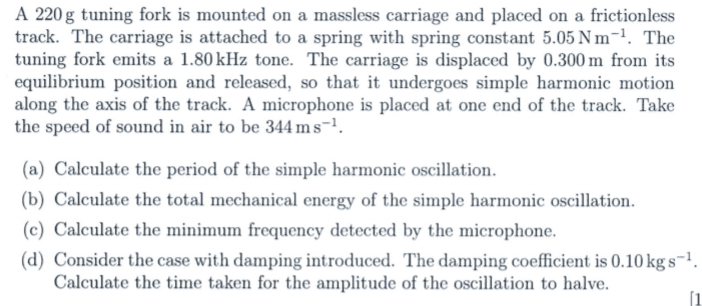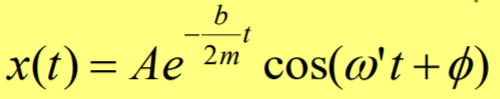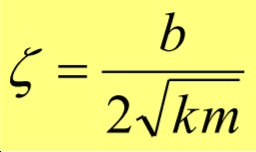# Simple harmonic motion - damping introduced

• Jozefina Gramatikova
In summary, the homework equation is ## A e^{-\frac{b}{2m} t}=\frac{1}{2} Ae^{-\frac{b}{2m} 0} ##, and the amplitude halves after 3.036 seconds.f

## Homework Statement## Homework Equations[/B]

## The Attempt at a Solution

#### Attachments

The damping coefficient is ## b ## in your formula. Do you know how to solve ## e^{-\frac{b}{2m}t}=\frac{1}{2} ## for ## t ## ? . ## \\ ## Edit: A google of the topic calls ## \gamma=\frac{b}{2m} ## the damping coefficient, but it is really a choice of terminology. By the units they give you, they are giving you ## b ##.

The damping coefficient is ## b ## in your formula. Do you know how to solve ## e^{-\frac{b}{2m}t}=\frac{1}{2} ## for ## t ## ? .
I don't know how much is x(t)

I don't know how much is x(t)
The sinusoidal oscillation is assumed to happen at a much higher frequency with small damping, so that the period of the oscillation ## T ## is quite short, and you don't need to consider the term ## \cos(\omega t ) ##. The amplitude is ## A e^{- \frac{b}{2m} t} ##.

•Jozefina Gramatikova
The sinusoidal oscillation is assumed to happen at a much higher frequency with small damping, so that the period of the oscillation ## T ## is quite short, and you don't need to consider the term ## \cos(\omega t ) ##. The amplitude is ## A e^{- \frac{b}{2m} t} ##.
Ok, thank you and what about x(t)

Your (first equation) that you posted in part 2 of the OP for ## x(t) ## is correct. (You didn't list an equation for ## \omega' ## though). And your second equation, which I think is a damping ratio number is really not of prime interest here. It would help if you would state what the parameter is. I had to go googling for the second equation you posted, to see if it was correct.## \\ ## When they say, how long does it take for the amplitude to "halve" that means ## A e^{-\frac{b}{2m} t}=\frac{1}{2} Ae^{-\frac{b}{2m} 0} ##, so that ## e^{-\frac{b}{2m} t}=\frac{1}{2} ##.

•Jozefina Gramatikova
Oh, I am sorry I didn't think an equation for w' would be helpful here. Thank you very much for your help!

•Now, the next step is take the natural log of both sides of this last equation in order to solve for ## t ##. (It may be worthwhile for me to mention that, because I'm not sure how advanced you may be).

Now, the next step is take the natural log of both sides of this last equation in order to solve for ## t ##. (It may be worthwhile for me to mention that, because I'm not sure how advanced you may be).
Yeah, I know how to proceed from here ## A e^{-\frac{b}{2m} t}=\frac{1}{2} Ae^{-\frac{b}{2m} 0} ##,. I got t=3.036s. I hope that this is correct

•••